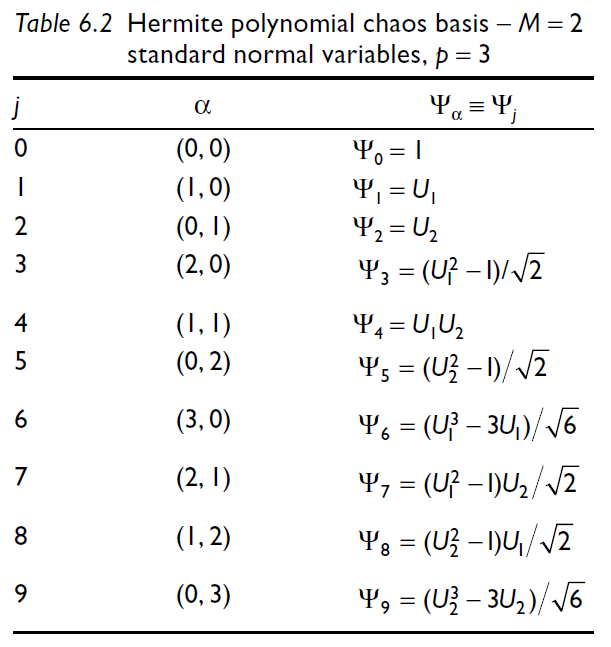# Correlation between the PCE coefficients and the indices of the PCE basis

Hi !

I have a not so simple question to ask…

I would like to know how uqlab associates the PCE coefficients with the indices in its basis.

Formulate it differently, I would like if UqLab follows the order that is presented in the book “RISK AND RELIABILITY IN GEOTECHNICAL ENGINEERING”, chapter 6, Tab.6.2, (written by M. Sudret) for the storing of the coefficients.I built a polynomial basis on another software and I try to use the coefficients calculated by UqLab and I have difficulties to match the coefficients with the right indices of my basis.

I know that my question is a bit out of the scope of other questions asked on the forum but I hope you can answer me anyway.

Best regards

I am just a UQlab user, so I do not know all the coding structure of the toolbox. However, I have been working with PCE and UQlab for a while now, so I try to give you an answer.You can check the degree of each univariate polynomial with the command

``````full(<yourPCEstruct>.PCE.Basis.Indices)
``````

As an example, you have a PCE computed for a model of dimensionality M=2 (as in the picture you just added). The indices will look like as

``````0 0    % average coefficient
0 1    % x_2
1 0    % x_1
0 2    % x_2^2
2 0    % ...
1 1
0 3
3 0
1 2
2 1
...
``````

If you want to recreate the same order of the Table you pasted, a simple permutation will do the job.1 Like

Hi !